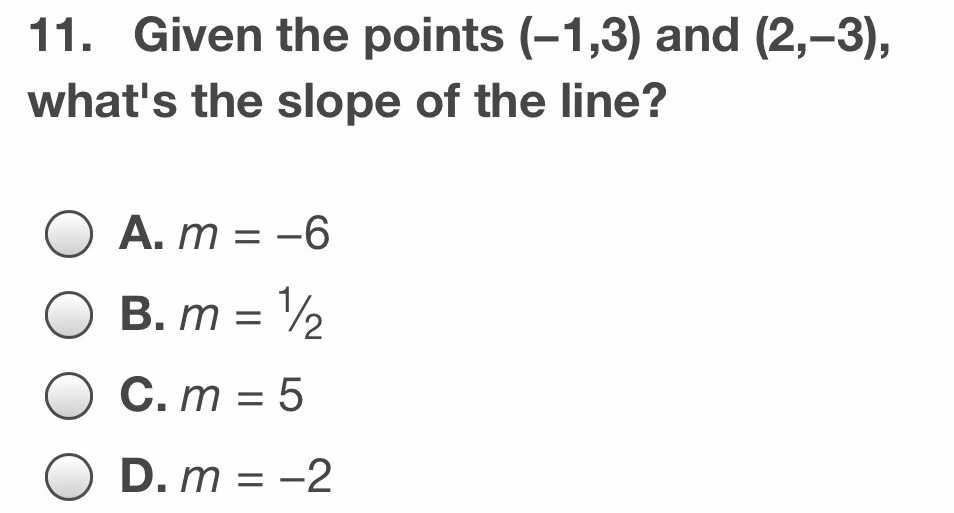### ¿Todavía tienes preguntas de matemáticas?

Pregunte a nuestros tutores expertos
Algebra
Pregunta11. Given the points $$( - 1,3 )$$ and $$( 2 , - 3 )$$ , what's the slope of the line?

A. $$m = - 6$$

B. $$m = 1 / 2$$

C. $$m = 5$$

D. $$m = - 2$$